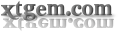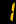" /> SIMONWAP_TODAY'S_PHYSICS EXAM
Pair of Vintage Old School FruYou Are Using A mobile Device
SIMONJar FUNNY TRIVIA!
EXAM-PORTAL SUPER QUOTES!
SIMON NIGERIA
ALWAYS POST WWW.EXAMPORTAL.XTGEM.COM TO YOUR FB,2GO,ESKIMI E.T.C STATUS
PHYSIC OBJ: 1-10: AACCCAABDC 11-20: BABAADAADD 21-30: DAAABBACDC 31-40: CDBDBACCAA 41-50: CCBADCCABD
(1a) Projectile is a body undergoing a motion under the combined effect of horizontal and vertical forces (1b) The horizontal components of the velocity remains the same because the body undergoes a uniform motion ========================= (2) Angle of projection (tita)=30degrees velocity(u)=150m/s At greatest height, v=0 t=Usin*tita/g t=(150*sin30degrees)/10 =150*0.5/10 t=7.5s ========================= (3) Given original lenght (Lo)=2.00m radius(r)=1.0mm=1*10^-3m Extension (e)=25mm=2*10^-3m Force(f)=10^3N stress =force/area Area(a)pie*R^2 =22/7*(10^-3)^2 3.142*10^16m^2 Stress=10^3/3.142*10^-6 =3.18*10^-1*10^3*10^6 =3.18*10^8Nm^-2 Strain=Extension/original length =25*10^-3/2 =1.25*10^-2 Young modulus (Y)=3.18*10^8/1.25*10^-2 Y-2.54*10^10Nm^-2 ========================= (4) -By wind -By increasing the cup surface area -By reducing the temperature ========================= (7a) De brogile equation states that landa=h/mv where landa=wavelenght of light mv=p=momentum h=planck's constant (7b) The equation is used to explain the wave-nature of matter ========================= (8ai) Uniform acceleration occurs when there is an equal changein n velocity with equal time interval no matter how small the time interval may be (8aii) V=wr V=linear velocity w=angular velocity r=radius (8b) R= resultant R=root(F1^2 F2^2) R=root(30^2 40^2) R=root(2500) R=50N (8c) This is done to increase the surface friction in water (8di) Given;mass(m)=20kg angle of inclineation (tita)=30degrees F=UR F=Umgcos*tita ma*sin*tita=Umg cos tita U=tan*tita U=tan30 U=0.51 (8dii) Frictional force(Fr) Fr=Umgcos*tita Fr=0.51*20*10*c0s30 Fr=90.9N (10ai) Resonance ia a phenomenon whereby a vibrating body sets another bodies vibrating all oscillating at d same neutal frequency (10aii) (1)Radio-recieving circuit (2)Sets of bob of pendulum (10aiii) Loudness is the sensation of sound reaching d ear while intensity is d ratio of d power to d area (10b) Wavelenght & speed (10d) Given; focal lenght(f)=18.0m Object distance(u)=90.0m Image height(hi)=1.0m (10di) 1/u+1/v=1/f 1/90+1/v=-1/18 1/v=-1/18-1/90 1/v=(-5-1)/90 1/v=-6/90 v=-90/6 =-15cm (10dii) v/u=hi/ho 15/90=1.0/h0 ho=90/15 =6cm Lenght of shark is 6cm (12a) Nucleon number is the sum of proton number and neutron number (12b) 95=c+a 95=c+2 c=95-2 c=93 (12bi) Number of neutrons in americium-241 is 237-93=144 (12ii) a=2, b=4, c=93 (12ci) Gamma ray is not deflected because it has no charge (12cii) -It has the highest penetrating power -it has low ionizing power (12d) N/Nt=(1/2)^t1/2/T 1/32=(1/2)^(110/t1/2) 5=110/t1/2 5t1/2=110 t1/2=110/5 t1/2=22s t1/2=0.693/landa 22=0.693/landa landa=0.693/22 landa=0.0315 landa=0.0315 per year
 Quick Menu FORUM BLOG CHATROOM WAPMASTER ZONE EXAM EXPO ZONE GUESTBOOK OYOBAZE.WAP-KA.COM ZUMAWAP.WAP-KA.COM JAVA THEMES XTGEM TOOLSWAPKA TOOLS ALL CODE ZONE
Share Page On Social NetworkOnline : 1 user
Today : 1391 user
Total all : 135968 Visitor
SIMON OFFICIAL WEBSITE
Mr SIMONjâr„¢